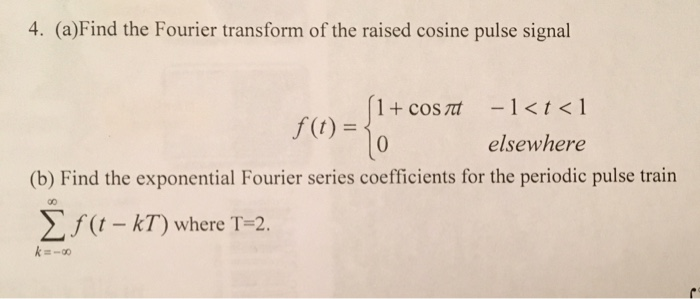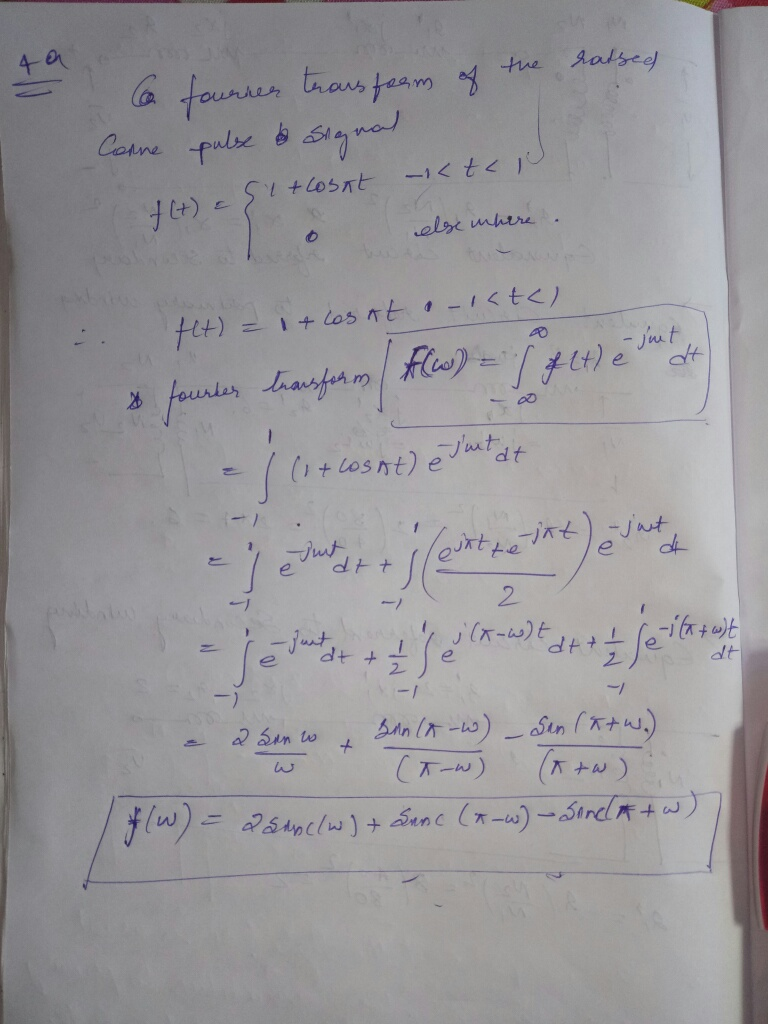# Homework Solution: Find the Fourier transform of the raised cosine pulse signal f(t) = {1 + cos pi t – 1…Find the Fourier transform of the raised cosine pulse signal f(t) = {1 + cos pi t - 1